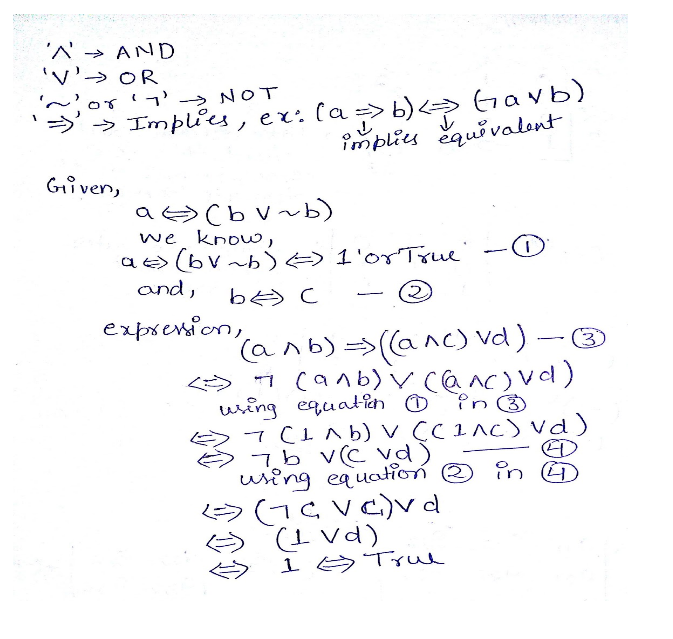Related Articles
GATE | GATE-CS-2000 | Question 30
• Difficulty Level : Medium
• Last Updated : 16 Dec, 2014

Let a, b, c, d be propositions. Assume that the equivalences a ↔ (b V-b) and b ↔ c hold. Then the truth value of the formula (a ∧ b) → (a ∧ c) ∨ d) is always
(A) True
(B) False
(C) Same as the truth value of b
(D) Same as the truth value of d

Answer: (A)

Explanation:

Notations (identities):

(aVb)⬄(a AND b)
(ab)⬄(a OR b)
(-a)⬄(NOT a)
(a=>b)⬄(aVb)This solution is contributed by Sandeep pandey.

Quiz of this QuestionMy Personal Notes arrow_drop_up